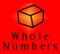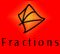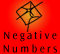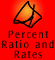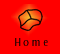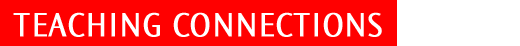| Teaching Connections for decimals | Which models to use? |
| Zero is tricky | Rounding | Addition | Subtraction | Multiplication and Division |

Teaching connections for decimals

We have covered the decimals topic on this CD-ROM in much the same way as would be done in the classroom. Below we have provided some further information on appropriate models to use and important concepts to reinforce before attempting operations on decimals.

For a more comprehensive look at some approaches to teaching decimals, ideas for lessons, common misconceptions and other useful information, please see the decimals website which is currently situated at, http://www.edfac.unimelb.edu.au/DSME/decimals/

It is unnecessary to do operations with common fractions before decimals, however children do need to know the relationship between common fractions and decimals.

For example 1/10 = 0.1, 1/100 = 0.01

Decimals with tenths can be introduced in about Year 3. Other place values can be introduced subsequently but care must be taken to ensure that decimal numbers with a range of place values are covered concurrently. For example, you would not want to spend too long working solely with hundredths. It is best to work with, say, tenths and hundredths at the same time, once both are learnt. In the early to middle years children can count in quarters and halves and so should effectively be able to count in tenths. This implies they should be able to do additions such as 0.2 + 0.3 informally.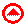Which models to use?

Linear Arithmetic Blocks

Linear Arithmetic Blocks, LAB, are a powerful model for decimals because the blocks can be arranged in a linear fashion. MAB is often the standard choice of model, however its application is not quite as obvious because the quantities are represented by volume rather than length. There is also potential for confusion with MAB as it has probably already been used to introduce whole numbers and renaming of blocks needs to occur, i.e. ones, tens, hundreds would need to be renamed as hundredths, tenths and ones.

 One Tenth Hundredth Thousandth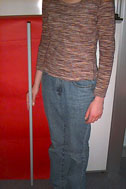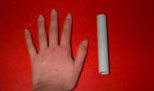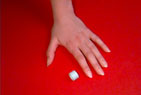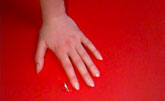LAB cannot be bought commercially but it is easy to make. See the Decimals Project website (address given above) for more details.

MAB (Multi-base Arithmetic Blocks)

It is common to use MAB to illustrate decimal ideas as well as whole number ideas. So, the MAB flat (or block) is assigned the value 1 and the other pieces represent tenths, hundredths (and thousandths). We do not recommend this, because students find the change in value confusing.

Number Expander

Although not a model as such, the number expander is a great visual aid which clearly shows various representations of expanded notation.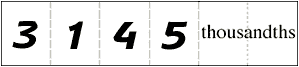Measurement models

Measurement models, such as the metric system and our decimal monetary system, are good support models for decimals but are not sufficient on their own. The range of places used in both systems is limited. In the metric system units of length such as millimetres, centimetres, decimetres and metres are best applied. The decimal currency system also has the drawback that people often think of dollars and cents as parallel units, i.e., they do not think of cents as fractions of a dollar.

Area Model

Another possible model for decimals is the area model. The area model consists of a unit square divided into 10 rows and 10 columns, where 1 cell represents 0.01, one row or column represents 0.1 and the unit square represents 1. With a coloured version of this model, children can easily see that ten hundredths is equivalent to 1 tenth and that 10 tenths is equivalent to 1 unit.

Templates are provided on the Decimals Project website which allow you to print and cut paper (or card) and then use area to illustrate decimal numbers. Once again, the benefit of this model over, say MAB, is that the students have probably not used it before and will therefore be unlikely to confuse the decimal version with representation of whole numbers.Zero is tricky!

Children need to understand:

• the superfluous role of the zero at the end of a decimal number. For example 3.7 = 3.70

• that zero in other places within a number does change the value of the number

• that 0 = 0.0 = 0.00 = 0.000 etc. They need to understand that all of these representations of zero are less than all the positive numbers.

For example, a common misconception is that 0 is greater than 0.6.

In order to avoid these problems, it is very important that children gradually build up the idea of one number line which holds all real numbers, otherwise children may have the misconception that decimals fit on one number line, whole numbers on another and fractions on another. Children need to see that all these numbers can be accommodated on the one number line.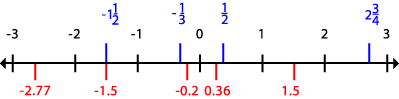Rounding

Rounding numbers can be illustrated well with LAB. Children need to understand that the purpose of rounding numbers is to find a number which is close to another number. Children who have trouble rounding numbers usually do not understand the meaning of decimal numbers.

For example, a child might think that 0.435 is not close to 0.4 because 435 is not close to 4.

The context for rounding a number must be clear. The degree of accuracy required in rounding always depends on the context.

For example, if we are talking about flying in a jet aeroplane we talk in kilometres, say, it is 150 kilometres to our destination. If the plane was taxiing on the runway it is more appropriate to talk in metres, say, the plane is about 100 metres from the end of the runway.

Example: If a car is travelling at 100 km/h what distance is it travelling in 1 second?

100 km/hr ÷ 3600 seconds = 0.027777777.. km

This can be converted to 27.777777.. metres, where '.77777..' goes on infinitely. However this answer does not really make sense. 28 metres would be more appropriate since the 100 km/h was probably not exact anyway.

In this example we can see that calculations can introduce a false level of accuracy.

Example: If Georgie aims to save \$35 per month to eventually save \$4000 for a holiday, how many months will it take?

114.2875 months is the answer produced on my calculator, Yet it is sufficient, and more meaningful, to say a bit over 114 months. 0.2875 of a month is not easily translated into a portion of time off the top of our heads.

 These examples show that the context and the sense of the answer are very important. Children can get stuck focusing on what they think is the right or most accurate answer while losing sight of what they are really trying to find out.Before attempting any operations on decimal numbers, children need to have a very clear understanding of the meaning of decimals. Addition of ragged decimals, i.e. decimals with varying numbers of decimal places (for example, 6.5314 + 8.2) requires a solid understanding of equivalent decimals. Children must be able to express decimals with various place values as the unit. For example, expressing 0.451 in terms of tenths, hundredths or thousandths.

When working with ragged decimals, children can initially use zeros to make the decimal numbers the same length and when sufficiently familiar they can use labelled place value columns. Children may also choose to place zeros in labelled place value columns. There are movies using labelled place value columns in the Addition of Decimals and Subtraction of Decimals sections of the CD-ROM.

A common teacher error is to think of the decimal operations as parallel to the whole number operations and therefore fairly easy for children to understand. However children encounter conceptual difficulties when working with decimal operations rather than difficulties actually applying the algorithms.Subtraction

To avoid errors children need to put in zeros in ragged decimals and then apply the appropriate algorithm. (This practice is then continued by most adults.)Multiplication and Division

It is not worth trying to use concrete materials extensively when teaching multiplication and division of decimals. Although materials can illustrate the meaning of some simple questions such as 2 ÷ 0.4 they do not adequately illustrate the algorithms. The algorithms are best understood by working with properties of numbers. We are trying to teach children to make the links so at some point materials need to be left behind.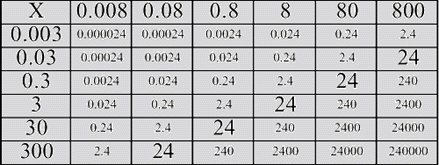Looking at the chart we can see the effect of dividing and multiplying by factors of 10. Children need to come to understand these patterns, such as multiplying one factor by 10 whilst dividing the other factor by 10 gives the same answer.

The inverse relationship between multiplication and division must be reinforced from whole number work. If children understand that division is the inverse of multiplication, then they can see that a division problem can be changed to a multiplication problem.

A common misconception is for children to think that division of two numbers will always produce a smaller number. Children need to work with many examples to see the patterns that evolve, i.e. when dividing by a number between 0 and 1 the answer will be larger.

2 ÷ 0.4 = 5

0.8 ÷ 0.4 = 2

0.8 ÷ 2 = 0.4

The number slide is another visual tool/anchor which can be used to show the results of multiplying and dividing decimal numbers by multiples of ten.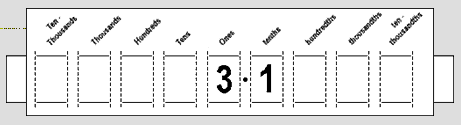Specifically it can show that:

• it is not the decimal point that moves, the digits do

• in order to multiply or divide by 10 we do not need to do the algorithm, we can see the digits change their place value column

• which zeros matter in representing the number.

Example: 2.76 x 10 = 27.6

The number slide can be used to show this answer and therefore demonstrate that the rule of adding zero does not work in this case.

Example:

304 x 10 = 3040

304 ÷ 10 = 30.4

30.4 ÷ 10 = 3.04

30.40 ÷ 10 = 3.04

By moving the number slider we can see which zeros matter.